# NCERT Solutions: Mathematical Reasoning Notes | Study Mathematics (Maths) Class 11 - JEE

## JEE: NCERT Solutions: Mathematical Reasoning Notes | Study Mathematics (Maths) Class 11 - JEE

The document NCERT Solutions: Mathematical Reasoning Notes | Study Mathematics (Maths) Class 11 - JEE is a part of the JEE Course Mathematics (Maths) Class 11.
All you need of JEE at this link: JEE

NCERT QUESTION  - (Mathematical Reasoning)

Question 1: Which of the following sentences are statements? Give reasons for your answer.

(i) There are 35 days in a month.

(ii) Mathematics is difficult.

(iii) The sum of 5 and 7 is greater than 10.

(iv) The square of a number is an even number.

(v) The sides of a quadrilateral have equal length.

(vii) The product of (–1) and 8 is 8.

(viii) The sum of all interior angles of a triangle is 180°.

(ix) Today is a windy day.

(x) All real numbers are complex numbers.

ANSWER :   (i) This sentence is incorrect because the maximum number of days in a month is 31. Hence, it is a statement.

(ii) This sentence is subjective in the sense that for some people, mathematics can be easy and for some others, it can be difficult. Hence, it is not a statement.

(iii) The sum of 5 and 7 is 12, which is greater than 10. Therefore, this sentence is always correct. Hence, it is a statement.

(iv) This sentence is sometimes correct and sometimes incorrect. For example, the square of 2 is an even number. However, the square of 3 is an odd number. Hence, it is not a statement.

(v) This sentence is sometimes correct and sometimes incorrect. For example, squares and rhombus have sides of equal lengths. However, trapezium and rectangles have sides of unequal lengths. Hence, it is not a statement.

(vi) It is an order. Therefore, it is not a statement.

(vii) The product of (–1) and 8 is (–8). Therefore, the given sentence is incorrect. Hence, it is a statement.

(viii) This sentence is correct and hence, it is a statement.

(ix) The day that is being referred to is not evident from the sentence. Hence, it is not a statement.

(x) All real numbers can be written as a × 1 +  0 × i. Therefore, the given sentence is always correct. Hence, it is a statement.

Question 2: Give three examples of sentences which are not statements. Give reasons for the answers.

ANSWER :   The three examples of sentences, which are not statements, are as follows.

(i) He is a doctor.

It is not evident from the sentence as to whom ‘he’ is referred to. Therefore, it is not a statement.

(ii) Geometry is difficult.

This is not a statement because for some people, geometry can be easy and for some others, it can be difficult.

(iii) Where is she going?

This is a question, which also contains ‘she’, and it is not evident as to who ‘she’ is. Hence, it is not a statement.

Question 3: Write the negation of the following statements:

(i) Chennai is the capital of Tamil Nadu.

(ii)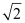is not a complex number.

(iii) All triangles are not equilateral triangle.

(iv) The number 2 is greater than 7.

(v) Every natural number is an integer.

(ii)is a complex number.

(iii) All triangles are equilateral triangles.

(iv) The number 2 is not greater than 7.

(v) Every natural number is not an integer.

Question 4: Are the following pairs of statements negations of each other?

(i) The number is not a rational number.

The number x is not an irrational number.

(ii) The number x is a rational number.

The number x is an irrational number.

ANSWER :   (i) The negation of the first statement is “the number x is a rational number”.

This is same as the second statement. This is because if a number is not an irrational number, then it is a rational number.

Therefore, the given statements are negations of each other.

(ii) The negation of the first statement is “the number x is not a rational number”. This means that the number x is an irrational number, which is the same as the second statement.

Therefore, the given statements are negations of each other.

Question 5: Find the component statements of the following compound statements and check whether they are true or false.

(i) Number 3 is prime or it is odd.
(ii) All integers are positive or negative.
(iii) 100 is divisible by 3, 11 and 5.

ANSWER :   (i) The component statements are as follows.

p: Number 3 is prime.

q: Number 3 is odd.

Both the statements are true.

(ii) The component statements are as follows.

p: All integers are positive.

q: All integers are negative.

Both the statements are false.

(iii) The component statements are as follows.

p: 100 is divisible by 3.

q: 100 is divisible by 11.

r: 100 is divisible by 5.

Here, the statements, p and q, are false and statement r is true.

Question 6: For each of the following compound statements first identify the connecting words and then break it into component statements.

(i) All rational numbers are real and all real numbers are not complex.

(ii) Square of an integer is positive or negative.

(iii) The sand heats up quickly in the Sun and does not cool down fast at night.

(iv) = 2 and x = 3 are the roots of the equation 3x2 – x – 10 = 0.

ANSWER :   (i) Here, the connecting word is ‘and’.

The component statements are as follows.

p: All rational numbers are real.

q: All real numbers are not complex.

(ii) Here, the connecting word is ‘or’.

The component statements are as follows.

p: Square of an integer is positive.

q: Square of an integer is negative.

(iii) Here, the connecting word is ‘and’.

The component statements are as follows.

p: The sand heats up quickly in the sun.

q: The sand does not cool down fast at night.

(iv) Here, the connecting word is ‘and’.

The component statements are as follows.

p: x = 2 is a root of the equation 3x2 – x – 10 = 0

qx = 3 is a root of the equation 3x2 – x – 10 = 0

Question 7: Identify the quantifier in the following statements and write the negation of the statements.

(i) There exists a number which is equal to its square.

(ii) For every real number xx is less than x + 1.

(iii) There exists a capital for every state in India.

ANSWER :   (i) The quantifier is “There exists”.

The negation of this statement is as follows.

There does not exist a number which is equal to its square.

(ii) The quantifier is “For every”.

The negation of this statement is as follows.

There exist a real number x such that x is not less than x + 1.

(iii) The quantifier is “There exists”.

The negation of this statement is as follows.

There exists a state in India which does not have a capital.

Question 8: Check whether the following pair of statements is negation of each other. Give reasons for the answer.

(i) xy  + x is true for every real numbers x and y.

(ii) There exists real number x and y for which x  + yy + x.

ANSWER :   The negation of statement (i) is as follows.

There exists real number x and y for which xy y +  x. This is not the same as statement (ii).

Thus, the given statements are not the negation of each other.

Question 9: State whether the “Or” used in the following statements is “exclusive “or” inclusive. Give reasons for your answer.

(i) Sun rises or Moon sets.

(ii) To apply for a driving licence, you should have a ration card or a passport.

(iii) All integers are positive or negative.

ANSWER :   (i) Here, “or” is exclusive because it is not possible for the Sun to rise and the moon to set together.

(ii) Here, “or” is inclusive since a person can have both a ration card and a passport to apply for a driving licence.

(iii) Here, “or” is exclusive because all integers cannot be both positive and negative.

Question 10: Rewrite the following statement with “if-then” in five different ways conveying the same meaning.

If a natural number is odd, then its square is also odd.

ANSWER :   The given statement can be written in five different ways as follows.

(i) A natural number is odd implies that its square is odd.

(ii) A natural number is odd only if its square is odd.

(iii) For a natural number to be odd, it is necessary that its square is odd.

(iv) For the square of a natural number to be odd, it is sufficient that the number is odd.

(v) If the square of a natural number is not odd, then the natural number is not odd.

Question 11: Write the contra positive and converse of the following statements.

(i) If x is a prime number, then x is odd.

(ii) It the two lines are parallel, then they do not intersect in the same plane.

(iii) Something is cold implies that it has low temperature.

(iv) You cannot comprehend geometry if you do not know how to reason deductively.

(v) x is an even number implies that x is divisible by 4

ANSWER :   (i) The contra positive is as follows.

If a number x is not odd, then x is not a prime number.

The converse is as follows.

If a number x is odd, then it is a prime number.

(ii) The contra positive is as follows.

If two lines intersect in the same plane, then they are not parallel.

The converse is as follows.

If two lines do not intersect in the same plane, then they are parallel.

(iii) The contra positive is as follows.

If something does not have low temperature, then it is not cold.

The converse is as follows.

If something is at low temperature, then it is cold.

(iv) The contra positive is as follows.

If you know how to reason deductively, then you can comprehend geometry.

The converse is as follows.

If you do not know how to reason deductively, then you cannot comprehend geometry.

(v) The given statement can be written as follows.

If x is an even number, then x is divisible by 4.

The contra positive is as follows.

If x is not divisible by 4, then x is not an even number.

The converse is as follows.

If x is divisible by 4, then x is an even number.

Question 12: Write each of the following statement in the form “if-then”.

(i) You get a job implies that your credentials are good.

(ii) The Banana trees will bloom if it stays warm for a month.

(iii) A quadrilateral is a parallelogram if its diagonals bisect each other.

(iv) To get A  in the class, it is necessary that you do the exercises of the book.

ANSWER :   (i) If you get a job, then your credentials are good.

(ii) If the Banana tree stays warm for a month, then it will bloom.

(iii) If the diagonals of a quadrilateral bisect each other, then it is a parallelogram.

(iv) If you want to get an A  in the class, then you do all the exercises of the book.

Question 13: Given statements in (a) and (b). Identify the statements given below as contrapositive or converse of each other.

(a) If you live in Delhi, then you have winter clothes.

(i) If you do not have winter clothes, then you do not live in Delhi.

(ii) If you have winter clothes, then you live in Delhi.

(b) If a quadrilateral is a parallelogram, then its diagonals bisect each other.

(i) If the diagonals of a quadrilateral do not bisect each other, then the quadrilateral is not a parallelogram.

(ii) If the diagonals of a quadrilateral bisect each other, then it is a parallelogram.

ANSWER :   (a) (i) This is the contrapositive of the given statement (a).

(ii) This is the converse of the given statement (a).

(b) (i) This is the contrapositive of the given statement (b).

(ii) This is the converse of the given statement (b).

Question 14: Show that the statement

p: “If x is a real number such that x3 + 4= 0, then x is 0” is true by

(i) direct method

(iii) method of contrapositive

ANSWER :   p: “If x is a real number such that x3 + 4x = 0, then x is 0”.

Let qx is a real number such that x3 + 4x = 0

rx is 0.

(i) To show that statement p is true, we assume that q is true and then show that r is true.

Therefore, let statement q be true.

∴ x3 + 4x = 0

(x2 + 4) = 0

⇒ x = 0 or x 4 = 0

However, since is real, it is 0.

Thus, statement r is true.

Therefore, the given statement is true.

(ii) To show statement p to be true by contradiction, we assume that p is not true.

Let x be a real number such that x3 + 4x = 0 and let x is not 0.

Therefore, x3 + 4x = 0

x (x2 + 4) = 0

x = 0 or x2 + 4 = 0

x = 0 or x2 = – 4

However, x is real. Therefore, = 0, which is a contradiction since we have assumed that x is not 0.

Thus, the given statement p is true.

(iii) To prove statement p to be true by contrapositive method, we assume that r is false and prove that q must be false.

Here, r is false implies that it is required to consider the negation of statement r. This obtains the following statement.

rx is not 0.

It can be seen that (x2 + 4) will always be positive.

x ≠ 0 implies that the product of any positive real number with x is not zero.

Let us consider the product of x with (x2 + 4).

∴ (x2 + 4) ≠ 0

⇒ x3 + 4x ≠ 0

This shows that statement q is not true.

Thus, it has been proved that

r ⇒ ∼q

Therefore, the given statement p is true.

Question 15: Show that the statement “For any real numbers a and ba2b2 implies that ab” is not true by giving a counter-example.

ANSWER :   The given statement can be written in the form of “if-then” as follows.

If a and b are real numbers such that a2b2, then ab.

Let pa and b are real numbers such that a2b2.

qab

The given statement has to be proved false. For this purpose, it has to be proved that if p, then ∼q. To show this, two real numbers, and b, with a2b2 are required such that a ≠ b.

Let a = 1 and b = –1

a2 = (1)2 = 1 and b2 = (– 1)2 = 1

∴ a2b2

However, a ≠ b

Thus, it can be concluded that the given statement is false.

Question 16: Show that the following statement is true by the method of contrapositive.

pIf x is an integer and x2 is even, then x is also even.

ANSWER :   p: If x is an integer and x2 is even, then x is also even.

Let qx is an integer and x2 is even.

rx is even.

To prove that p is true by contrapositive method, we assume that r is false, and prove that q is also false.

Let x is not even.

To prove that q is false, it has to be proved that x is not an integer or x2 is not even.

x is not even implies that x2 is also not even.

Therefore, statement q is false.

Thus, the given statement p is true.

Question 17: By giving a counter example, show that the following statements are not true.

(i) p: If all the angles of a triangle are equal, then the triangle is an obtuse angled triangle.

(ii) q: The equation x2 – 1 = 0 does not have a root lying between 0 and 2.

ANSWER :   (i) The given statement is of the form “if q then r”.

q: All the angles of a triangle are equal.

r: The triangle is an obtuse-angled triangle.

The given statement p has to be proved false. For this purpose, it has to be proved that if q, then ∼r.

To show this, angles of a triangle are required such that none of them is an obtuse angle.

It is known that the sum of all angles of a triangle is 180°. Therefore, if all the three angles are equal, then each of them is of measure 60°, which is not an obtuse angle.

In an equilateral triangle, the measure of all angles is equal. However, the triangle is not an obtuse-angled triangle.

Thus, it can be concluded that the given statement p is false.

(ii) The given statement is as follows.

q: The equation x2 – 1 = 0 does not have a root lying between 0 and 2.

This statement has to be proved false. To show this, a counter example is required.

Consider x2 – 1 = 0

x2 = 1

x = ± 1

One root of the equation x2 – 1 = 0, i.e. the root x = 1, lies between 0 and 2.

Thus, the given statement is false.

Question 18: Which of the following statements are true and which are false? In each case give a valid reason for saying so.

(i) p: Each radius of a circle is a chord of the circle.

(ii) q: The centre of a circle bisects each chord of the circle.

(iii) r: Circle is a particular case of an ellipse.

(iv) s: If and y are integers such that xy, then –x < –y.

(v) t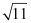is a rational number.

ANSWER :   (i) The given statement p is false.

According to the definition of chord, it should intersect the circle at two distinct points.

(ii) The given statement q is false.

If the chord is not the diameter of the circle, then the centre will not bisect that chord.

In other words, the centre of a circle only bisects the diameter, which is the chord of the circle.

(iii) The equation of an ellipse is,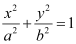If we put ab = 1, then we obtain

x2y2 = 1, which is an equation of a circle

Therefore, circle is a particular case of an ellipse.

Thus, statement r is true.

(iv) y

⇒ –x < –y (By a rule of inequality)

Thus, the given statement s is true.

(v) 11 is a prime number and we know that the square root of any prime number is an irrational number. Therefore,is an irrational number.

Thus, the given statement t is false.

Question 19: Write the negation of the following statements:

(i) p: For every positive real number x, the number x – 1 is also positive.

(ii) q: All cats scratch.

(iii) r: For every real number x, either x > 1 or x < 1.

(iv) s: There exists a number x such that 0 < x < 1.

ANSWER :   (i) The negation of statement p is as follows.

There exists a positive real number x, such that x – 1 is not positive.

(ii) The negation of statement q is as follows.

There exists a cat that does not scratch.

(iii) The negation of statement r is as follows.

There exists a real number x, such that neither x > 1 nor x < 1.

(iv) The negation of statement s is as follows.

There does not exist a number x, such that 0 < x < 1.

Question 20: State the converse and contrapositive of each of the following statements:

(i) p: A positive integer is prime only if it has no divisors other than 1 and itself.

(ii) q: I go to a beach whenever it is a sunny day.

(iii) r: If it is hot outside, then you feel thirsty.

ANSWER :   (i) Statement p can be written as follows.

If a positive integer is prime, then it has no divisors other than 1 and itself.

The converse of the statement is as follows.

If a positive integer has no divisors other than 1 and itself, then it is prime.

The contrapositive of the statement is as follows.

If positive integer has divisors other than 1 and itself, then it is not prime.

(ii) The given statement can be written as follows.

If it is a sunny day, then I go to a beach.

The converse of the statement is as follows.

If I go to a beach, then it is a sunny day.

The contrapositive of the statement is as follows.

If I do not go to a beach, then it is not a sunny day.

(iii) The converse of statement r is as follows.

If you feel thirsty, then it is hot outside.

The contrapositive of statement r is as follows.

If you do not feel thirsty, then it is not hot outside.

Question 21: Write each of the statements in the form “if p, then q”.

(i) p: It is necessary to have a password to log on to the server.

(ii) q: There is traffic jam whenever it rains.

(iii) r: You can access the website only if you pay a subscription fee.

ANSWER :   (i) Statement p can be written as follows.

If you log on to the server, then you have a password.

(ii) Statement q can be written as follows.

If it rains, then there is a traffic jam.

(iii) Statement r can be written as follows.

If you can access the website, then you pay a subscription fee.

Question 22: Re write each of the following statements in the form “p if and only if q”.

(i) p: If you watch television, then your mind is free and if your mind is free, then you watch television.

(ii) q: For you to get an A grade, it is necessary and sufficient that you do all the homework regularly.

(iii) r: If a quadrilateral is equiangular, then it is a rectangle and if a quadrilateral is a rectangle, then it is equiangular.

ANSWER :   (i) You watch television if and only if your mind is free.

(ii) You get an A grade if and only if you do all the homework regularly.

(iii) A quadrilateral is equiangular if and only if it is a rectangle.

Question 23: Given below are two statements

p: 25 is a multiple of 5.

q: 25 is a multiple of 8.

Write the compound statements connecting these two statements with “And” and “Or”. In both cases check the validity of the compound statement.

ANSWER :   The compound statement with ‘And’ is “25 is a multiple of 5 and 8”.

This is a false statement, since 25 is not a multiple of 8.

The compound statement with ‘Or’ is “25 is a multiple of 5 or 8”.

This is a true statement, since 25 is not a multiple of 8 but it is a multiple of 5.

Question 24: Check the validity of the statements given below by the method given against it.

(i) p: The sum of an irrational number and a rational number is irrational (by contradiction method).

(ii) q: If n is a real number with n > 3, then n2 > 9 (by contradiction method).

ANSWER :   (i) The given statement is as follows.
p: the sum of an irrational number and a rational number is irrational.

Let us assume that the given statement, p, is false. That is, we assume that the sum of an irrational number and a rational number is rational.

Therefore,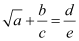, where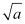is irrational and bcde are integers.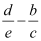is a rational number andis an irrational number.

This is a contradiction. Therefore, our assumption is wrong.

Therefore, the sum of an irrational number and a rational number is rational.

Thus, the given statement is true.

(ii) The given statement, q, is as follows.

If n is a real number with n > 3, then n2 > 9.

Let us assume that n is a real number with n > 3, but n2 > 9 is not true.

That is, n2 < 9

Then, n > 3 and n is a real number.

Squaring both the sides, we obtain

n2 > (3)2

⇒ n2 > 9, which is a contradiction, since we have assumed that n2 < 9.

Thus, the given statement is true. That is, if n is a real number with n > 3, then n2 > 9.

Question 25: Write the following statement in five different ways, conveying the same meaning.

p: If triangle is equiangular, then it is an obtuse angled triangle.

ANSWER :   The given statement can be written in five different ways as follows.

(i) A triangle is equiangular implies that it is an obtuse-angled triangle.

(ii) A triangle is equiangular only if it is an obtuse-angled triangle.

(iii) For a triangle to be equiangular, it is necessary that the triangle is an obtuse-angled triangle.

(iv) For a triangle to be an obtuse-angled triangle, it is sufficient that the triangle is equiangular.

(v) If a triangle is not an obtuse-angled triangle, then the triangle is not equiangular.

The document NCERT Solutions: Mathematical Reasoning Notes | Study Mathematics (Maths) Class 11 - JEE is a part of the JEE Course Mathematics (Maths) Class 11.
All you need of JEE at this link: JEEUse Code STAYHOME200 and get INR 200 additional OFF

## Mathematics (Maths) Class 11

154 videos|171 docs|132 tests

Track your progress, build streaks, highlight & save important lessons and more!

,

,

,

,

,

,

,

,

,

,

,

,

,

,

,

,

,

,

,

,

,

;